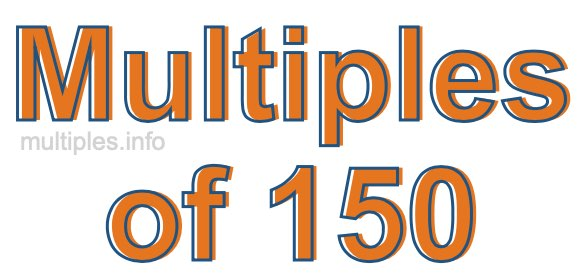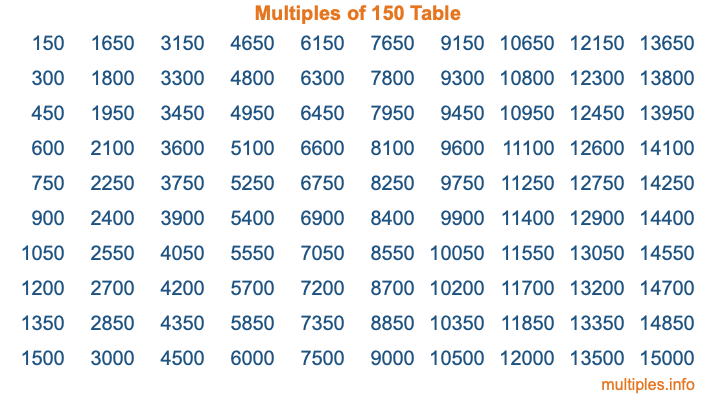Multiples of 150Welcome to the Multiples of 150 page. Here we will first teach you everything you will ever need to know about the multiples of 150, and then give you a study guide summary of everything we taught you to make sure you remember it all. Use this page to look up facts and learn information about the multiples of 150. This page will make you a multiples of one hundred fifty expert!

Definition of Multiples of 150
Multiples of 150 are all the numbers that when divided by 150 equal an integer. Each of the multiples of 150 are called a multiple. A multiple of 150 is created by multiplying 150 by an integer.

Therefore, to create a list of multiples of 150, you start with 1 multiplied by 150, then 2 multiplied by 150, then 3 multiplied by 150, and so on for as long as you want. Thus, the list of the first five multiples of 150 is 150, 300, 450, 600, and 750. To see a larger list of multiples of 150, see the printable image of Multiples of 150 further down on this page. We also have a category where you can choose any nth multiple of 150.

Multiples of 150 Checker
The Multiples of 150 Checker below checks to see if any number of your choice is a multiple of 150. In other words, it checks to see if there is any number (integer) that when multiplied by 150 will equal your number. To do that, we divide your number by 150. If the the quotient is an integer, then your number is a multiple of 150.

Is  a multiple of 150?

Least Common Multiple of 150 and ...
A Least Common Multiple (LCM) is the lowest multiple that two or more numbers have in common. This is also called the smallest common multiple or lowest common multiple and is useful to know when you are adding our subtracting fractions. Enter one or more numbers below (150 is already entered) to find the LCM.

Check out our LCM Calculator if you need more details about the Least Common Multiple or if you need the LCM for different numbers for adding and subtraction fractions.

nth Multiple of 150
As we stated above, 150 is the first multiple of 150, 300 is the second multiple of 150, 450 is the third multiple of 150, and so on. Enter a number below to find the nth multiple of 150.

th multiple of 150

Multiples of 150 vs Factors of 150
150 is a multiple of 150 and a factor of 150, but that is where the similarities end. All postive multiples of 150 are 150 or greater than 150. All positive factors of 150 are 150 or less than 150.

Below is the beginning list of multiples of 150 and the factors of 150 so you can compare:

Multiples of 150: 150, 300, 450, 600, 750, etc.

Factors of 150: 1, 2, 3, 5, 6, 10, 15, 25, 30, 50, 75, 150

As you can see, the multiples of 150 are all the numbers that you can divide by 150 to get a whole number. The factors of 150, on the other hand, are all the whole numbers that you can multiply by another whole number to get 150.

It's also interesting to note that if a number (x) is a factor of 150, then 150 will also be a multiple of that number (x).

Multiples of 150 vs Divisors of 150
The divisors of 150 are all the integers that 150 can be divided by evenly. Below is a list of the divisors of 150.

Divisors of 150: 1, 2, 3, 5, 6, 10, 15, 25, 30, 50, 75, 150

The interesting thing to note here is that if you take any multiple of 150 and divide it by a divisor of 150, you will see that the quotient is an integer.

Multiples of 150 Table
Below is an image of the first 100 multiples of 150 in a table. The table is in chronological order, column by column. The first column has the first ten multiples of 150, the second column has the next ten multiples of 150, and so on.The Multiples of 150 Table is also referred to as the 150 Times Table or Times Table of 150. You are welcome to print out our table for your studies.

Negative Multiples of 150
Although not often discussed or needed in math, it is worth mentioning that you can make a list of negative multiples of 150 by multiplying 150 by -1, then by -2, then by -3, and so on, to get the following list of negative multiples of 150:

-150, -300, -450, -600, -750, etc.

Multiples of 150 Summary
Below is a summary of important Multiples of 150 facts that we have discussed on this page. To retain the knowledge on this page, we recommend that you read through the summary and explain to yourself or a study partner why they hold true.

There are an infinite number of multiples of 150.

A multiple of 150 divided by 150 will equal a whole number.

150 divided by a factor of 150 equals a divisor of 150.

The nth multiple of 150 is n times 150.

The largest factor of 150 is equal to the first positive multiple of 150.

150 is a multiple of every factor of 150.

150 is a multiple of 150.

A multiple of 150 divided by a divisor of 150 equals an integer.

150 divided by a divisor of 150 equals a factor of 150.

Any integer times 150 will equal a multiple of 150.

Multiples of a Number
Here you can get the multiples of another number, all with the same attention to detail as we did for multiples of 150 on this page.

Multiples of
Multiples of 151
Did you find our page about multiples of one hundred fifty educational? Do you want more knowledge? Check out the multiples of the next number on our list!